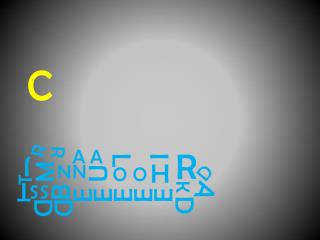Download PresentationMARIA VASALIS

# MARIA VASALIS - PowerPoint PPT PresentationDownload Presentation## MARIA VASALIS

- - - - - - - - - - - - - - - - - - - - - - - - - - - E N D - - - - - - - - - - - - - - - - - - - - - - - - - - -
##### Presentation Transcript

1. C R R A A R I L J M U H D N N O O T B A E E E E E T K S S D D D

2. C R R A A R I L J M U H D N N O O T B A E E E E E T K S S D D D

3. C R R A A R I L J M U H D N N O O T B A E E E E E T K S S D D D

4. C R R A A R I L J M U H D N N O O T B A E E E E E T K S S D D D

5. C R R A A R I L J M U H D N N O O T B A E E E E E T K S S D D D

6. C R R A A R I L J M U H D N N O O T B A E E E E E T K S S D D D

7. C R R A A R I L J M U H D N N O O T B A E E E E E T K S S D D D

8. C R R I R A A L R R A A R J I L H M U J D N N O O M U H D N N T O O T B E A E E E E T K S S B A E E E E E T K S S D D D D MARIA VASALIS

9. SEXY POWERPOINT PROJECT Bart Verswijvel – 2011 - EiEU